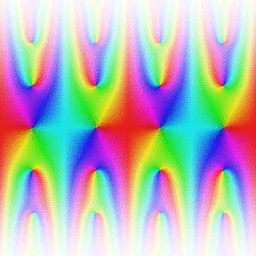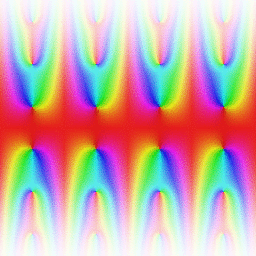# Theta functions and elliptic functions in Arb

October 21, 2014

Warning: this post contains graphical content that may cause elliptic seizures.

This is a followup to the previous post on modular forms in Arb. After some work, the modular forms module in Arb now supports:

• Modular transformations
• The Dedekind eta function $\eta(\tau)$
• The j-invariant $j(\tau)$
• The modular lambda function $\lambda(\tau)$
• The modular discriminant $\Delta(\tau)$
• The Eisenstein series $G_{2k}(\tau)$, for $k = 2, 3, 4, \ldots$
• The Jacobi theta functions $\theta_i(z,\tau)$, $i = 1,2,3,4$, with reduction of $\tau$ to the fundamental domain for rapid convergence
• The power series expansions with respect to $z$ of Jacobi theta functions (i.e. derivatives of any order)
• The Weierstrass elliptic function $\wp(z,\tau)$ and its series expansion with respect to $z$ to any order

The last bullet point basically means that Arb supports numerical evaluation of all elliptic functions, since the field of elliptic functions with fixed periods $1, \tau$ is generated by $\wp(z,\tau)$, $\wp'(z,\tau)$. In practice, there are many variations of the same elliptic functions, such as Jacobi's functions sn, cn, dn etc. These can all be computed from theta functions and their derivatives, even if not currently supported explicitly. Unfortunately, one often also needs inverse elliptic functions (i.e. elliptic integrals) and these are not yet supported.

As usual, the code should scale well. Here is how long it takes to simultaneously compute the first $N$ derivatives of all four theta functions at $z = \tau = 1+i$, with each derivative accurate to $N$ decimal digits (this requires a working precision of roughly $5.5N$ bits):

 N Time (seconds) 10 0.000037 100 0.0015 1000 0.15 10000 40

Now let's look at some pictures. Here is a plot of $\theta_1(z,\tau)$ with $\tau = 0.5+0.5i$:A fun thing to do with the plotting code is to output a sequence of images and join them into an animation. Here is an example with theta functions. Each frame shows $\theta_2(z,\tau)$ (left) and $\theta_3(z,\tau)$ (right) as a function of $z$ on $[-2,2] + [-2,2]i$ with $\tau$ fixed. We move the parameter $\tau$ along a sine curve path $\tau = 4t + (2/3 + \sin(\pi t)/3)i$ where $t$ is the time. The important features of the theta functions are illustrated nicely: the periodicity along the real axis, the exponential growth away from the real axis, the quasiperiodicity, the location of zeros, the absence of poles.I've discovered that staring at such animations for extended periods of time can be very interesting, especially in combination with the appropriate music. Your mileage may vary.

Here are a few (non-animated) portraits, of modular forms. The lambda function:The modular discriminant:The Eisenstein series $G_6$:The Eisenstein series $G_{22}$:I wish to clarify what I meant in the previous post when I said that modular forms are "fractals", since this offended people. This was not meant to imply that the geometric essence of a modular form is that it is fractal-like, or that a modular form even matches some particular formal notion of "fractal" (to begin with, a fractal is usually understood to be a set, not a function). The property I wanted to highlight is merely that if you draw a picture of a modular form in the upper half-plane and zoom in towards the real line, a similar pattern will repeat at any level of magnification.

Elliptic functions are, by definition, doubly periodic, and hence repeat the same pattern along a grid of parallelograms when plotted. Here is a picture of the Weierstrass $\wp$-function with $\tau$ fixed:I could make more pictures and movies, but I should probably get back to work...